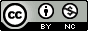## Tuesday, April 21, 2015

### R: single plot with two different y-axes

I forgot where I originally found the code to do this, but I recently had to dig it out again to remind myself how to draw two different y axes on the same plot to show the values of two different features of the data. This is somewhat distinct from the typical use case of aesthetic mappings in ggplot2 where I want to have different lines/points/colors/etc. for the same feature across multiple subsets of data.
For example, I was recently poking around with some data examining enrichment of a particular set of genes using a hypergeometric test as I was fiddling around with other parameters that included more genes in the selection (i.e., in the classic example, the number of balls drawn from some hypothetical urn). I wanted to show the -log10(p-value) on one axis and some other value (e.g., “n”) on the same plot, using a different axis on the right side of the plot.
Here’s how to do it. First, generate some data:
``````set.seed(2015-04-13)

d = data.frame(x =seq(1,10),
n = c(0,0,1,2,3,4,4,5,6,6),
logp = signif(-log10(runif(10)), 2))
``````
x n logp
1 0 1.400
2 0 0.590
3 1 1.200
4 2 1.500
5 3 0.028
6 4 0.380
7 4 2.500
8 5 0.067
9 6 0.041
10 6 0.360
The strategy here is to first draw one of the plots, then draw another plot on top of the first one, and manually add in an axis. So let’s draw the first plot, but leave some room on the right hand side to draw an axis later on. I’m drawing a red line plot showing the p-value as it changes over values of x.
``````par(mar = c(5,5,2,5))
with(d, plot(x, logp, type="l", col="red3",
ylab=expression(-log(italic(p))),
ylim=c(0,3)))
``````
Now, draw the second plot on top of the first using the `par(new=T)` call. Draw the plot, but don’t include an axis yet. Put the axis on the right side (`axis(...)`), and add text to the margin (`mtext...`). Finally, add a legend.
``````par(new = T)
with(d, plot(x, n, pch=16, axes=F, xlab=NA, ylab=NA, cex=1.2))
axis(side = 4)
mtext(side = 4, line = 3, 'Number genes selected')
legend("topleft",
legend=c(expression(-log(italic(p))), "N genes"),
lty=c(1,0), pch=c(NA, 16), col=c("red3", "black"))
``````

1.2.